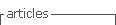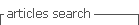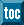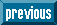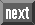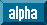## Indicators

•Cited by SciELO
•Access statistics

•Cited by Google
•Similars in SciELO
•Similars in Google

## Print version ISSN 0120-6230

### Rev.fac.ing.univ. Antioquia  no.76 Medellín July/Sept. 2015

#### http://dx.doi.org/10.17533/udea.redin.n76a17

ARTÍCULO ORIGINAL

First-principles calculations of the pressure dependence on the structural and electronic properties of GaN/CrN superlattice

Cálculos por primeros principios de la dependencia con la presión de las propiedades estructurales y electrónicas de la superred GaN/CrN

Miguel José Espitia-Rico*, John Hernán Díaz-Forero, Luis Eduardo Castillo-Méndez

Grupo de Estudio en temas de la Física, de la Estadística y la Matemática (GEFEM), Facultad de Ingeniería, Universidad Distrital Francisco José de Caldas. Carrera 7 N.° 40-53. Bogotá, Colombia.

* Corresponding author: Miguel José Espitia Rico, e-mail: mespitiar@udistrital.edu.co

ISSN 0120-6230

e-ISSN 2422-2844

(Received March 17, 2014; accepted May 14, 2015 )

Abstract

This work provides first-principle calculations to investigate how pressure affects the electronic and magnetic properties of 1x1-GaN/CrN superlattice. A method based on Full-Potential Linearized Augmented Plane Waves (FP-LAPW) is used exactly as implemented in code Wien2k. It was found that the most favorable phase for 1x1-GaN/CrN superlattice is the hexagonal wurtzite type, and also that, due to pressure, the superlattice can reach the NaCl phase, with a transition pressure PT1 = 13.5 GPa. Additionally, in the most favorable phase, it was observed that the magnetic moment changes from 0 to 2.1 µβ for a transition pressure PT2 = 25.50 GPa. From the calculations of the density states, it can be stated that the superlattice exhibits a half-metallic behavior at equilibrium pressure. Moreover, at high pressures P > PT2, the superlattice exhibits a metallic behavior. The evidence indicates that the superlattice may be used in spintronics.

Keywords: Electronic properties, pressure effects, superlattice

Resumen

En este trabajo se ejecutaron cálculos por primeros principios para investigar cómo la presión afecta las propiedades estructurales y electrónicas de la superred GaN/CrN. Se usó el método Ondas Planas Aumentadas y Linealizadas Potencial Completo (FP-LAPW), tal como está implementado en código Wien2k. Se encontró que la más favorable para la superred GaN/MnN es la hexagonal tipo wurtzita y que, debido a la presión, la superred puede pasar a la fase NaCl, siendo la presión de transición PT1 = 13,5 GPa. Adicionalmente, en la fase más favorable se halló que el momento magnético de la superred cambia, pasando de 0 a 2,1 µβ a la presión de transición PT2 = 26,50 GPa. Los cálculos de la densidad de estados demuestran que la superred posee un comportamiento semi-metálico a la presión de equilibrio y que, a presiones P > PT2, la superred posee un comportamiento metálico.

Palabras clave: Propiedades electrónicas, efectos de la presión, superred

1. Introduction

The Chromium nitride CrN has been extensively studied, both theoretically and experimentally, due to its excellent mechanical, physical and chemical properties (e.g. high hardness, high melting point, high resistance to wearing conditions, corrosion and oxidation) [1-3]. On the other hand, GaN is a direct-gap semiconductor that normally crystallizes in wurtzite [4, 5]. Due to its excellent properties, GaN has been used in a range of applications like radiation emitting and sensing devices as well as in high-power/high-temperature electronics; moreover, its efficiency in blue, green and yellow LEDs, injection lasers and ultraviolet detectors is extraordinary [6, 7]. Furthermore, the combination of the semiconductor properties of GaN and the magnetic properties of the transition metal ions is of great current interest due to the potential applications in diluted magnetic semiconductors (DMS) [8, 9].

Such an interest is also placed on various applications in spintronics (e.g. electro-optical switches and spin injectors) . In this paper we study the effects of pressure on the structural and magnetic properties of GaN/CrN superlattice. It is found that the magnetic moment of superlattice GaN/CrN changes from 0 to 2,1 μβ at pressure PT2 = 26.50 GPa. The magnetic moment is found to have a value of 3.0 μβ at equilibrium pressure and the superlattice exhibits a semi-metallic behavior, which suggests that the superlattice may be used in spintronics.

2. Computational method

The Calculations were performed within the framework of Density Functional Theory (DFT) and the Full Potential Augmented Plane Wave (FP-LAPW) was used as implemented in software package Wien2k . The exchange and correlation effects of the electrons are dealt with using the generalized gradient approximation (GGA) of Perdew, Burke and Ernzerhof (PBE) . In the LAPW method, the cell is divided into two types of regions, namely spheres centered at the atomic nuclear sites and an interstitial region between non-overlapping areas. Within the atomic spheres, wave functions are replaced by atomic functions; whereas in the interstitial region, the function is expanded in the form of plane waves. The charge density and potential expand to form spherical harmonics up to lmax = 10 inside the atomic spheres, and the wave function in the interstitial region expands in the form of plane waves with a cutoff parameter RMT Kmax = 8, where RMT is the smallest radius of the atomic level within the unit cell and Kmax is the magnitude of the largest k vector of the reciprocal lattice. To ensure convergence in the integration of the first Brillouin zone, 1600 points were used, which corresponds to 140 k-points at the irreducible part of the first Brillouin zone for the NaCl phase, and 144 k-points at the irreducible part of the first Brillouin zone for the wurtzite phase.

The integrals over the Brillouin zone are solved using the special approximation of k points provided by Monkhorst-Pack. Self-consistency is achieved by requiring that the convergence of the total energy is less than 10-4 Ry. To achieve expansion of the potential in the interstitial region, Gmax = 12 is considered. The corresponding muffin-tin radii were 1,6 bohr for N, 1.95 bohr for Ga and 1.85 for Cr. Calculations were performed considering the spin polarization caused by the presence of chromium in the superlattice.

In order to determine the most stable crystallization phase at finite pressure and temperature values as well as the possible crystal phase transition caused by pressure, the Gibbs free energymight be used. Since the calculations are in ground state (T = 0 ° K), the last term of the Gibbs energy can be neglected; and by working with the enthalpy, Gibbs free energy reduces to H = E + PV [13-15]. We use this equation for the two crystal structures considered in this work; however, we cannot exclude the possible existence of other stable or metastable structures for GaN/CrN superlattice.

To calculate the lattice constant, the minimum volume, the bulk modulus and the cohesive energy of the two structures studied, calculations were adjusted to the Murnaghan equation of state (Eq. 1)Where B0 is the bulk modulus, its first derivative is B'0, V0 is equilibrium volume of the cell, and E0 represents the cohesive energy.

3. Results

3.1 Structural properties

The GaN/CrN superlattice modeled in the NaCl phase, by interleaving a layer of GaN and a layer of CrN along the z axis, crystallizes in a tetragonal structure with space group 123 (P4/mmm) . On the other hand, the superlattice modeled in wurtzite phase crystallizes in a structure that belongs to space group 156 (P3m1) . The calculated values for the lattice constants of the superlattice, c/a​​, the minimum volume (V0), the bulk modulus (B0) and the cohesive energy (E0) (per formula unit) of the GaN/CrN superlattice in the wurtzite and NaCl structures, are shown in Table 1.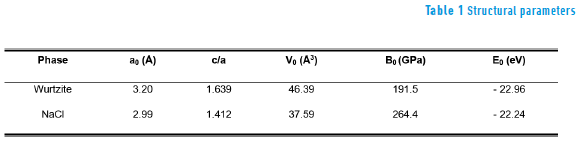It can be observed that the smallest value of minimum energy E0 occurs in the wurtzite phase; therefore, the most favorable crystallization structure for the 1x1 GaN/CrN superlattice is the hexagonal wurtzite type. The values ​​of the bulk modules are high, confirming that this superlattice exhibits high hardness, which makes it attractive for potential applications at high temperatures and in hard coatings.

The Figure 1 shows the cohesive energy curves as a function of volume. These curves have been adjusted according to the Murnaghan equation of state (Eq. 1) for each of the two structural phases considered in this study. The values for energy and volumes are given per formula unit of GaN/CrN superlattice.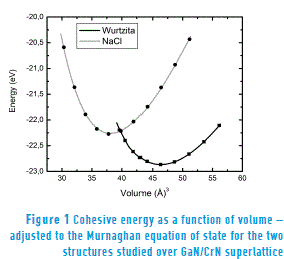Figure 1 also shows that the curve representing the most stable wurtzite-type hexagonal phase intersects the curve of the NaCl structure with the smallest equilibrium volume. This indicates that there is a structural phase transition of the superlattice from the Wurtzite phase to the NaCl phase. The minimum energy E0 of the GaN/CrN superlattice in the NaCl phase is 0.216 eV/(formula unit); this is higher than the corresponding energy for the wurtzite phase, which prevents spontaneous changes of phase. Therefore, the phase transition in the superlattice must be induced by applying external pressure. In order to describe the phase transition, enthalpy is calculated as a function of pressure for each structure. By overlaying the resulting enthalpy lines as a function pressure, as shown in Figure 2, we obtain a value of PT1 = 13.5 GPa for the transition pressure.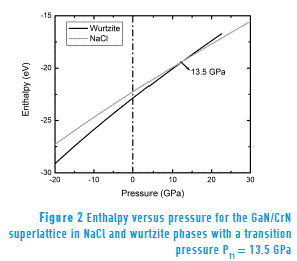Note that, before the transition (P < PT1), the lowest enthalpy value corresponds to wurtzite phase, indicating relative stability of this phase for the pressure range in question. On the other hand, after the transition (P > PT1), the NaCl phase exhibits the lowest enthalpy value, confirming its stability in this region of the phase diagram.

3.2 Electronic properties

Initially, for the most stable phase (i.e. the hexagonal wurtzite type), we calculate the variation of the magnetic moment as a function of the lattice constant (and therefore as a function of the pressure). The findings suggest that the magnetic moment of the superlattice changes from 0 to 2.1 μβ at a transition pressure PT2 ∼ 26.50 GPa (a = 3.11 Å), indicating that the pressure affects the magnetic properties of the superlattice. Additionally, we found that, at the equilibrium pressure P = 0, the magnetic moment of the GaN/CrN superlattice reaches a constant value of 3.0 μβ; this value is attributed to the electron configuration of Cr3 +, where atoms of Cr ferromagnetically couple to GaN .

To analyze the effects of pressure on the electronic properties of the GaN/CrN superlattice, we calculate the density of electronic states for the spin-up and spin-down polarizations of the GaN/CrN superlattice. Figure 3 shows the total and partial state density calculated with the equilibrium lattice constant (therefore at equilibrium pressure P = 0). It can be observed that, near the Fermi level, the GaN/CrN superlattice becomes conductive for the spin-up density. This is mainly determined by the Cr-d states and, to a lesser extent, by the N-p electrons. On the other hand, the superlattice becomes a semiconductor for spin-down density (no spin-down contribution). Therefore, the superlattice exhibits a half-metallic behavior at P = 0.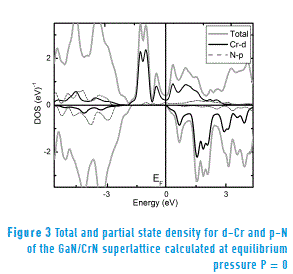The Figure 4 shows the state density calculated at high pressure (namely 35 GPa, which exceeds the transition pressure, P > PT2 = 26.5 GPa). In both, the valence band and the conduction band, there is spin-up and spin-down polarization; therefore, due to the effect of pressure, the superlattice loses its half-metallic properties and shows a metallic behavior. A similar result was obtained in the study using DFT in GaxV1 - xN compounds .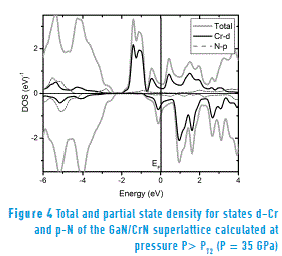Finally, for both the state density at equilibrium pressure (Figure 3) and the state density calculated at a higher pressure to the transition pressure (P = 35 GPa > PT2 = 26.50 GPa) (Figure 4) are determined mainly by the Cr-d and N-p hybrid states that cross the Fermi level. Moreover, according to the theory proposed for the bond between metal Cr-d and non-metal N-p states , results in a covalent bonding and such bonding positively contributes to hardness, which explains the high hardness of GaN/CrN superlattice.

4. Conclusions

In this work, we used first principles to analyze how pressure affects the structural and electronic properties of GaN/CrN superlattice. We found that, the most favorable phase of crystallization for the superlattice is the wurtzite-type hexagonal phase; but due to pressure, the superlattice can reach the NaCl phase from the wurtzite structure, with transition pressure PT1 = 13.5 GPa. The magnetic moment changes for a transition pressure PT2; in particular, we found that the magnetic moment changes from 0 to 2.1 μβ at to PT2 = 26.5 GPa. The magnetic moment has a value of 3.0 μβ at equilibrium pressure P = 0. At the equilibrium pressure, the GaN/CrN superlattice has a half-metallic behavior and such a behavior is mainly determined by the Cr-d electrons and, to a lesser extent, by N-p states. On the basis of this study, it follows that the spin polarization is dependent on pressure and may be manipulated experimentally. Due this properties the GaN/CrN superlattice is a good alternative when choosing a ferromagnetic material for applications in spintronics or spin injectors.

5. Acknowledgment

The authors would like to thank the Research Centre at Universidad Distrital Francisco José de Caldas for the financial support in this project.

6. References

1.  A. Zaoui, S. Kacimi, B. Bouhafs, A. Roula. "First-principles study of bonding mechanisms in the series of Ti, V, Cr, Mo, and their carbides and nitrides".Physica B: Condesed Matter. Vol. 358. 2005. pp. 63-71.         [ Links ]

2.  Z. Dridi, B Bouhafs, P. Ruterana, H. Aourag. "First-principles calculations of vacancy effects on structural and electronic properties of TiCx and TiNx". Journal Physic Condensed Matter. Vol. 14. 2002. pp. 10237-10249.         [ Links ]

3.  I. Pollini, A. Mosser, J. Parlebas. "Electronic, spectroscopic and elastic properties of early transition metal compounds". Physics Reports. Vol. 355. 2001. pp.1-72.         [ Links ]

4.  O. Arbouche, B. Belgoumène, B. Soudini, M. Driz. "First principles study of the relative stability and the electronic properties of GaN". Computational Materials Science. Vol. 47. 2009. pp. 432-438.         [ Links ]

5.  J. Wagner, F. Bechstedt. "Properties of strained wurtzite GaN and AlN: Ab initio studies". Physical Review B. Vol. 66. 2002. pp. 115202(1)-115202(20).         [ Links ]

6.  S. Nakamura, M. Senoh, S. Nagahama, N. Iwasa, T. Yamada, T. Matsushita, et al. "Room-temperature continuous-wave operation of InGaN multi-quantum-well structure laser diodes with a lifetime of 27 hours". Appl. Phys. Lett. Vol. 70. 1997. pp. 1417-1421.         [ Links ]

7.  S. Nakamura, M. Senoh, N. Iwasa, S. Nagahama. "High‐power InGaN single‐quantum‐well‐structure blue and violet light‐emitting diodes". Applied Physics Letters. Vol. 67. 1995. pp. 1868-1871.         [ Links ]

8.  H. Bar, S. Zamir, O. Katz, B. Meyler, J. Salzman. "GaN layer growth optimization for high power devices". Materials Science and Engineering A. Vol. 302. 2001. pp. 14-17.         [ Links ]

9.  K. Sato, P. Dederics, H. Katayama. "Curie temperatures of III-V diluted magnetic semiconductors calculated from first principles". Europhysics Letters. Vol. 61. 2003. pp. 403-408.         [ Links ]

10.  A. Houari, S. Matar, M. Belkhir. "Stability and magnetic properties of Mn-substituted ScN semiconductor from first principles". Computational Materials Science. Vol. 43. 2008. pp. 392-398.         [ Links ]

11.  P. Blaha, K. Schwarz, S. Trickey. "Electronic Structure of solids whit WIEN2k". Molecular Physics. Vol. 108. 2010. pp. 3147-3166.         [ Links ]

12.  J. Perdew, K. Burke, M. Ernzerhof. "Generalized gradient approximation made simple". Physical Review Letter. Vol. 77. 1996. pp. 3865-3868.         [ Links ]

13.  R. González, W. López, J. Rodríguez. "First-principles calculations of structural properties of GaN-V". Solid State Communications. Vol. 144. 2007. pp. 109-113.         [ Links ]

14.  L. Mancera, J. Rodríguez, N. Takeuchi. "Theoretical study of the stability of wurtzite, zinc-blende, NaCl and CsCl phases in group IIIB and IIIA nitrides". Phys. Status Solid B. Vol. 241. 2004. pp. 2424-2429.         [ Links ]

15.  N. Takeuchi. "First-principles calculations of the ground-state properties and stability of ScN". Physical Rewiew B. Vol. 65. 2002. pp. 1-4.         [ Links ]

16.  L. Yanlu, F. Weiliu, S. Honggang, C. Xiufeng, Z. Xian, J. Minhua. "Nitrogen vacancy and ferromagnetism in Cr-doped GaN: First-principles calculations". Journal of Solid State Chemistry. Vol. 183. 2010. pp. 2662-2668.         [ Links ]

17.  M. Espitia, C. Ortega, G. Casiano. "Multicapa TiN/GaN: un estudio ab initio". Revista colombiana de Física. Vol. 43. 2011. pp. 408-410.         [ Links ]

18.  M. Espitia, C. Ortega, J. Díaz. "Theoretical study of the structural and electronic properties of the ScxCr1-xN compound". Revista Latinoamericana de Metalurgia y Materiales. Vol. 36. 2015. pp. 12-19.         [ Links ]

19.  R. González, W. López, F. Fajardo, J. Rodríguez. "Pressure effects on the electronic and magnetic properties of GaxV1-xN compounds: Ab-initio study". Materials Science and Engineering B. Vol. 163. 2009. pp. 190-193.         [ Links ]

20.  S. Jhi, J. Ihm, S. Louie, M. Cohen. "Electronic mechanism of hardness enhancement in transition-metal carbonitrides". Nature. Vol. 399. 1999. pp. 132-134.         [ Links ]